```|Portada|Blog|Space|
```
```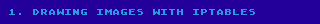To understand what I'm talking about here, I *really* suggest watching
this video titled: "Harder Drive: Hard drives we didn't want or need":

which at the 7:46 it says: "and it wouldn't be that hard to embed some
kind of picture or message in here, like how cool would it be to embed
a little qr code in this... right? it'd be a little bit cool... maybe
a little embarrassing too.  Anyway, that's the Internet."

This means that for this blog post we are going to paint the following
picture of me, taken in the Korankei gorge, located in Asuke-chō, Aichi,
Japan.  Yes, I know, the background is not very recognizable.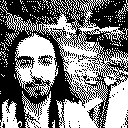So, the trivial solution would be to convert each black or white pixel
into a rule in our router's firewall, for example to draw a black pixel:
iptables -A \$some_chain -d \$pixel_ip -j DROP

However this would not only require at least 7839 rules for this
small image making our firewall huge and slow, but would completely lack
any fun, and so in the spirit of a Harder Drive it makes sense to find an
overly complicated way to achieve the same result.

Btw, to convert the IP into the coordinates of the image, we can use the
transformation already mentioned on the video, called Hilbert curve:
https://en.wikipedia.org/wiki/Hilbert_curve
and so I'm going to steal the implementation written there.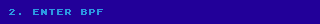Did you know iptables supports a module called "bpf"?

This bpf module allows us to associate a small program (a small sequence of
byte-codes) to a rule, that will be run for each packet to determine if it
matches or not.  Even though its computation model is not Turing complete,
it give us a great lot of flexibility.

To write bpf programs we have basically two alternatives, one of them is
using the pcap library directly to write the compile it from a pcap
expression, either by using tcpdump on a raw interfaces such as tun*, or
with a script like this one (changing «code» in the first line to specify
the program you would like to compile):

\$ luajit -e 'code = "dst 10.71.1.1"
local ffi = require "ffi"
ffi.cdef [[
struct bpf_insn { uint16_t code; uint8_t jt; uint8_t jf; uint32_t k; };
struct bpf_program { unsigned int bf_len; struct bpf_insn *bf_insns; };
int pcap_compile_nopcap(int, int, struct bpf_program *,
const char *, int, uint32_t);]]
prog = ffi.new "struct bpf_program"
assert(ffi.load "pcap".pcap_compile_nopcap(-1, 12, prog, code, 1, -1) == 0)
local t = {prog.bf_len}
for i = 0, prog.bf_len-1 do
local ins = prog.bf_insns[i]
t[#t+1] = ("%d %d %d %d"):format(ins.code, ins.jt, ins.jf, ins.k)
end
print(table.concat(t,","))'
7,48 0 0 0,84 0 0 240,21 0 3 64,32 0 0 16,21 0 1 172425473,6 0 0 4294967295,6 0 0 0

Here is the documentation for pcap/tcpdump expressions:

-> https://www.tcpdump.org/manpages/pcap-filter.7.html

And so to disassemble bpf byte-code you can use this program:

-> https://github.com/cloudflare/bpftools/blob/master/linux_tools/bpf_dbg.c

Let's then disassemble the program we just compiled:

\$ ./bpf_dbg
> load bpf 7,48 0 0 0,84 0 0 240,21 0 3 64,32 0 0 16,21 0 1 172425473,6 0 0 4294967295,6 0 0 0
> disassemble
l0:     ldb 
l1:     and #0xf0
l2:     jeq #0x40, l3, l6
l3:     ld 
l4:     jeq #0xa470101, l5, l6
l5:     ret #0xffffffff
l6:     ret #0

It makes sense right?
* l0 loads the first byte of the IP packet into the register A,
this byte contains the IP version in the upper nibble.
* l1 discards the lower nibble: it's doing a bit-wise and: A = A & 0xf0
* l2 compares the register A with the immediate value 0x40 jumping
to l3 if it is equal, or l6 otherwise.
* l3 loads the word (32 bits) at offset 16 which in an IP packet it is
the destination address, into the register A.
* l4 compares the register A with the immediate value 0x0A470101 which
is the IP 10.71.1.1 written in hex, if it matches it jumps to l5,
if it doesn't, it matches to l6.
* l5 returns -1, which by being different to 0 it means "match packet"
* l6 returns 0, meaning "don't match packet".

In other words, as expected the program "dst 10.71.1.1" matches a given
packet only if it has IP version 4, and if its destination IP address is
10.71.1.1.

The other alternative, which we will be using today, is to write bpf
programs in assembly and compile them using an assembler called "bpfc",
which is included in the netsniff-ng debian package.

In order to do this we need some knowledge about how the BPF model works.

* We have two registers, A and X.  A the accumulator can be used for
computations, and the X register can be used for indirect addressing.
* There is a scratch memory bank composed of 16 32bit registers M to
M, which doesn't support indexed addressing and can only be used
on the instructions: ld, ldx, st, stx for transferring to and fro the
registers A and X.
* Arithmetic and logic operations will always use A as the left operand
and destination register, while the right operand can either be a 32-bit
immediate value or the register X:
a := a «op» #imm
a := a «op» x
The following operations are provided: arithmetic: add, sub, mul, div, mod;
logic: or, and, lsh, rsh, xor; unary: neg (basically A := -A).
* Only jumps forward are allowed, the destination address is always a
relative immediate unsigned offset: This is important because ensures all
programs will terminate in at most as many steps as its size; no, there's
no call stack nor any kind of branch and link BL instruction.  There are no
functions/subroutines at all.
* Programs can finish by returning a constant (ret #imm) or the value of the
register A (ret a).  A return value of zero will not match the packet.
* Furthermore the maximum size allowed for BPF programs can be quite small as
we will see in the following section.

If you want more detail about the classic BPF, here is the paper with a good
introduction to BPF (it's a nice reading material), and the reference manual:

-> https://www.tcpdump.org/papers/bpf-usenix93.pdf
-> https://www.freebsd.org/cgi/man.cgi?bpf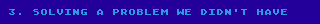So... how much space do we have?: 64, yes only 64 instructions... 😞

-> https://git.netfilter.org/iptables/tree/include/linux/netfilter/xt_bpf.h?id=18c96821b5901ac5c66dcbc5f299bd07ef5569ef#n8

Let's see, we can at least store 32 bits in a single immediate value, which
we can use by indexing it with the lowest 5 bits of the IP address, in C it
would be: (SOME_CONSTANT >> (address & 0x1f)) & 1.  This in BPF can be
programmed as:
l0: ld      // a := destination ip address
l1: and #0x1f   // a := a & 0x1f, leaving a number between 0 and 31
l2: tax         // x := a, copy into x so that we can shift right by it
l3: ld #BITSET  // a := BITSET, basically the value for 32 pixels
l4: rsh x       // a := a >> x, discards the lowest x bits
l5: and #1      // a := a & 1, leaving only the selected bit.
l6: ret a       // finish the program with either 1 or 0

To see how a right shift «rsh» can be used for indexing a bit, we can see these
two examples: if the IP address in binary ends with 00000, at l2: x will be set
to 0, and then the shift at l4 will not modify the BITSET value at register a,
meaning that l5 will select the lowest bit.

If the IP address on the other hand ends with 11111, at l2: x=31 (11111 in
binary is 31 in decimal), then the shift at l4 will shift the register A 31
bits to the right, and after the «and» at l5, only 0 or 1 will remain, it
being the content of the highest bit of BITSET.

So, how can we use more bits of the IP address to select what constant to use?
In any normal programming language we would normally just provide a table of
constants, like:

const uint32_t MY_TABLE[] = {
0x7fcbece9, 0xfffff7ff, 0xf7fffdff, 0xff7d777b,
0xfed7cfff, 0xfdfffbef, 0x639ff99f, 0x600d10f2,
0x9ffc0021, 0x8800bffc, 0x23858602, 0xffffff47,
0xefffffff, 0xffffffde, 0xffffffee, 0xebb3dffd };

and that would be the end of the problem, however there's no way to do this
in BPF, yes, we have an array of 16 memory locations, but there's no indexed

What we can do is to have a tree of branches!  There's a very useful
instruction called «jset» that does (a & «imm») jumping to a given location
if the value is non-zero and to another given location if it is zero, this
means that we can build a binary tree to jump to a specific «ld #BITSET», so
for 2 bits it can be:

jset #0x00000040, L1,  L0
L0:     jset #0x00000080, L01, L00
L1:     jset #0x00000080, L11, L10
L00:    ld #BITSET00
jmp l4
L01:    ld #BITSET01
jmp l4
L10:    ld #BITSET10
jmp l4
L11:    ld #BITSET11

And for N additional bits, this code would take 3*(2^N)-1 instructions, meaning
that for N=5 we would have 95 instructions, going over our total limit of 64.
For N=4 instead we have 47 instructions, plus the 6 we had before, we are well
within the margin.  In the end we've managed to check against 9 bits (4 for the
binary tree + 5 by right shifting a 32 bits constant with the lowest 5 bits of
the address), and we still have some additional space to check the remaining
32-9=23 high bits of the IP address against a constant, by starting the
program with, with in the end a grand total of 56 instructions:
ld 
jeq #NETWORK, ok
ret #0

To encode a 128*128 pixels image 2^14 pixels, we would then need 2^(14-9)=32
lines of iptables, something completely doable.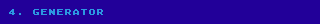I just include the generator for sake of completeness, it's certainly not a
polished piece of code, but it does the job:

#!/usr/bin/env luajit
local bit = require 'bit'

-- I run this program as follows:
--   ./bpfimg.lua 127.42.0.0/18 yo.pbm | sudo bash
-- and to check the generated assembly:
--   ./bpfimg.lua -d 127.42.0.0/18 yo.pbm
local function parse_args(arg)
local i = 1
local debug = false
if arg[i] == '-d' then
debug = true
i = i + 1
end
-- We parse the first argument as the network/mask we are matching:
local addr_str, cidr_str = arg[i]:match '^(%d+%.%d+%.%d+%.%d+)/(%d+)\$'
local network = 0
local n = tonumber(octet)
assert(0 <= n and n <= 255)
network = network * 256 + n
end)
local cidr = tonumber(cidr_str)
assert(18 <= cidr and cidr <= 32)
assert(cidr % 2 == 0, 'Images must have natural aligment')
local side = 2^((32 - cidr)/2)
local npixels = side * side

local imgdata
do
local fd = assert(io.open(arg[i+1]))
local rawdata = fd:read '*a':gsub('#.-\n', '')
local width, height, data = rawdata:match '^P1%s+(%d+)%s+(%d+)%s+(.*)'
imgdata = data:gsub('%s', '')
assert(imgdata, 'Image must be in XBM format')
assert(tonumber(width) == side and tonumber(height) == side,
'Expected square image of side ' .. side)
assert(#imgdata == width * height)
fd:close()
end
return network, cidr, side, npixels, imgdata, debug
end

local network, cidr, side, npixels, imgdata, debug = parse_args(arg)

-- This code is basically the C code at Wikipedia's article on Hilbert
-- curve translated to lua, this is why it's so "bit." heavy.
local function d2xy(d)
-- parameter d and return value are all 0-based.
local x, y, s = 0, 0, 1
while s < 65536 do
local rx = bit.band(1, bit.rshift(d, 1))
local ry = bit.band(1, bit.bxor(d, rx))
if ry == 0 then
if rx == 1 then
x = s-1 - x
y = s-1 - y
end
x, y = y, x
end
x = x + s * rx
y = y + s * ry
s = bit.lshift(s, 1)
d = bit.rshift(d, 2)
end
return x, y
end

-- Find corner with lowest values.  This could be optimized if we were
-- to abuse the fact that d2xy uses the Hilbert curve.
local ox, oy = d2xy(network)
for d = network, network + npixels-1 do
local x, y = d2xy(d)
ox, oy = math.min(x, ox), math.min(y, oy)
end

local function test_d2xy()
local visited = {}
local x, y = d2xy(network)
local px, py = x-1, y
for d = 0, npixels-1 do
x, y = d2xy(d + network)
assert(0 <= x-ox and x-ox < side)
assert(0 <= y-oy and y-oy < side)
assert(not visited[y*65536+x])
visited[y*65536+x] = true
assert(math.abs(x-px) + math.abs(y-py) == 1)
px, py = x, y
end
end
test_d2xy()

local genbits_visited = {}
-- Given an IP address as an integer number, calculate the bitset for
-- the 32 IPs block that the BPF code uses for the 5 LSb of the address
local function genbits(base)
local ret = 0
if base < 0 then base = base + 0x100000000 end
for i = 0, 31 do
local ip = base + i
local rel = ip - network
if 0 <= rel and rel < npixels then
local x, y = d2xy(ip)
-- we have to obtain the position in the XBM relative to the origin
local pos = (y - oy) * side + (x - ox) + 1
-- 49 is ('1'):byte(), representing the black color = DROP
if imgdata:byte(pos) == 49 then
-- LSb corresponds to the first IP in the range, since the bpf
-- code does: (ret >> (IP&31)) & 1
ret = ret + bit.lshift(1, i)
end
end
end
-- just to report some statics at the end:
local visit_count = genbits_visited[ret] or 0
if visit_count > 0 then
genbits_visited.duplicates = (genbits_visited.duplicates or 0) + 1
end
genbits_visited.total = (genbits_visited.total or 0) + 1
genbits_visited[ret] = visit_count + 1
return ret
end

local function toipstring(ip)
return ('%d.%d.%d.%d'):format(
bit.rshift(ip, 24),
bit.band(bit.rshift(ip, 16), 255),
bit.band(bit.rshift(ip, 8), 255),
bit.band(ip, 255))
end

-----------------------------------------------------------------------
-- Code generation!
-----------------------------------------------------------------------

-- If you run: sudo tcpdump -ilo -d 'dst host 127.0.0.1' you will see that the
-- generated code begins by checking the ETHERTYPE field on the ethernet frame
-- In iptables however the ethernet headers are not provided
-- (man 8 iptables-extensions):
--   > Iptables passes packets from the network layer up, without mac layer.

local chain_name = ('drop_icmp_%s/%d'):format(toipstring(network), cidr)

local function generate_rule(ip, cidr)
if cidr < 23 then
generate_rule(ip, cidr+1)
return generate_rule(ip + bit.lshift(1, 32-cidr-1), cidr+1)
end
-- we print the comment in cyan:
print(('# %srule for %s/%d%s'):format(
'\27[36m', toipstring(ip), cidr, '\27[0m'))
local prog = {}
local function out(s) prog[#prog+1] = s end
-- We don't really have to check the version, as IPv6 goes through ip6tables
out '  ld '

-- Then we check the top bits to ensure we are in the correct network

out('  jeq #0x' .. bit.tohex(ip) .. ', ok')
out '  ret #0'

-- let's load the dst ip again, this time to filter the last 5 bits only
out 'ok:'
out '  ld '
out '  and #31'
-- and save it in register x
out '  tax'

-- Now let's generate the tree for filtering the bits betwen cidr and 26
-- inclusive.  If there are no bits to test (cidr >= 27) then we just
-- load the immediate bits for band(network, 0xffffffe0)

if cidr >= 27 then
prog[#prog+1] = '  ld #0x' .. bit.tohex(genbits(bit.band(ip, 0xffffffe0)))
else
local function dump_tree(label, ip, cidr)
out(('%s:'):format(label))
-- we check against 32-5=27 to interleave the constants with the tree
-- and so theoretically support a larger jump tree if the number of
-- maximum instructions would be larger, like 2048, as all jumps would
-- fall within the 8-bit limit for jump offsets.
if cidr == 27 then
out(('  ld #0x%s'):format(bit.tohex(genbits(ip))))
if not label:find '^L_1+\$' then
out '  jmp finish'
end
else
local b = bit.lshift(1, 32-cidr-1)
out(('  jset #0x%s, %s1, %s0'):format(bit.tohex(b), label, label))
dump_tree(label..'0', ip, cidr+1)
dump_tree(label..'1', ip + b, cidr+1)
end
end
dump_tree('L_', ip, cidr)
end

out 'finish:'
out '  rsh x'  -- a = a >> x, with x being the last 5 bits of dst addr
out '  and #1'
out '  ret a'
io.write('iptables -A ' .. chain_name .. ' -m bpf --bytecode "')
local fd = assert(io.popen('bpfc -p -f xt_bpf -i -' ..
(debug and ' -V' or ''), 'w'))
fd:write(table.concat(prog, '\n'))
fd:close()
print '" -j DROP'
end

-- this header makes sure that our chain does not filter anything but pings:
print(('iptables -N %s || iptables -F %s'):format(chain_name, chain_name))
print('iptables -A '..chain_name.." '!' -p icmp -j RETURN")
print('iptables -A '..chain_name.." -p icmp '!' --icmp-type ping -j RETURN")
generate_rule(network, cidr)

-- there was some space for a bit more optimization
print(('# total=%d, duplicated=%d'):format(
genbits_visited.total, genbits_visited.duplicates))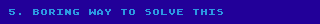If for some reason you need to have a dense set of IP addresses, then please
by all means just use ipset with type "bitmap:ip" or "hash:ip" (if the set is
sparse), or any of the additional types for other use cases.

If you need more complex stuff, you probably should use eBPF instead of BPF.

Maybe in the future I could write a second installment with a tutorial for
doing exactly this, but by writing a super efficient eBPF program instead.

.utterances {
margin-left: 0px;
filter: brightness(90%);
max-width: 640px;
transform-origin: top left;
transform: scale(.8);
}
```
```document.title="wiki: blog/drawing-with-iptables-harder-drive-followup"
```